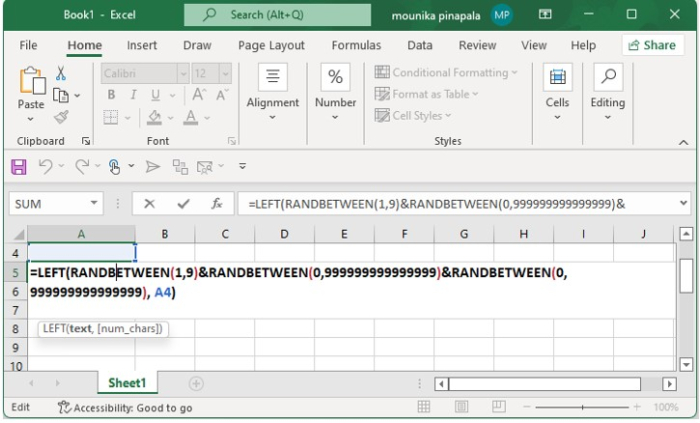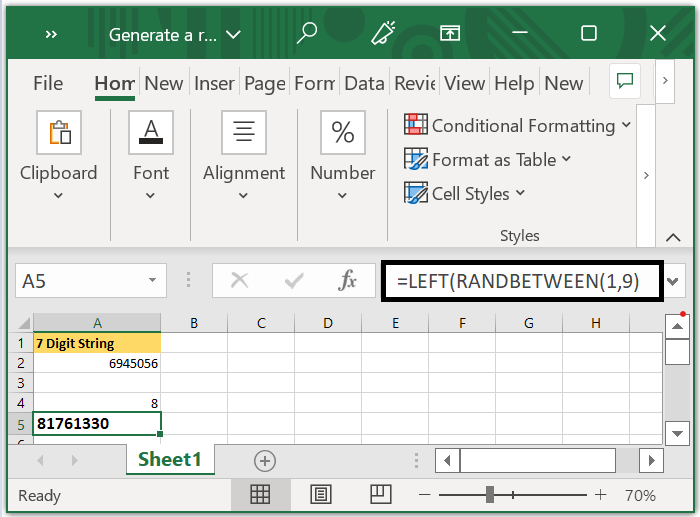# Generate a random number with the given specific length in Excel

Nowadays most of them use multiple passwords in our day-to-day work. The password may contain different lengths. If you are not willing to set a password then you can use formulas or a tool that is handy to generate random passwords for you. This article gives you some tricks to generate random numbers of your desired length in an Excel workbook.

### Random Number in a given specific length using Formula

Random number in a fixed length

Assume that you want to generate a random number string in a 7-digit length using a formula for this you can use the below formula.

 =RANDBETWEEN(1000000,9999999)Click on Enter key to get the 7-digit length as an output.

Kindly note that the output value will be changed every time when you update the Excel workbook.

Random number in a changed length based on the digit number that you type in another cell

For instance, if you want to generate the passwords in different lengths. Type 6 in the cell A4 and enter the below formula in the cell A5 and click on enter it will display a random number in 6-digit length and if you type 9 in the cell A4 with the same formula in cell A5 and clicking on enter it will display a random 9-digit length number. Below is the formula that you need to enter.

 =LEFT(RANDBETWEEN(1,9)&RANDBETWEEN(0,999999999999999)&RANDBETWEEN(0,999 999999999999), A4)

Now select A5 in the Excel workbook and enter the above formula.Now press enter key, A4 cell will display the blank cell or empty cell.

Enter a number 8 in the cell A4 and the same above-mentioned formula in the cell A5 and click on enter key. Then the cell A4 display the 8-digit length as shown in the below screenshot.In this way you can choose the number you desire to get the random number.

Kindly note that the numbers will be changed when ever you update the Excel worksheet.

This how you will generate random numbers in an Excel workbook using the formulas.